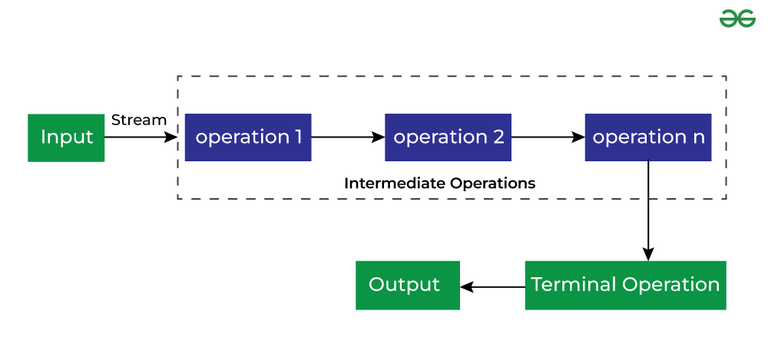Open In App

# Stream In Java

Introduced in Java 8, Stream API is used to process collections of objects. A stream in Java is a sequence of objects that supports various methods which can be pipelined to produce the desired result.

### Use of Stream in Java

There uses of Stream in Java are mentioned below:

1. Stream API is a way to express and process collections of objects.
2. Enable us to perform operations like filtering, mapping,reducing and sorting.

## How to Create Java Stream?

Java Stream Creation is one of the most basic steps before considering the functionalities of the Java Stream. Below is the syntax given on how to declare Java Stream.

### Syntax

`Stream<T> stream;`

Here T is either a class, object, or data type depending upon the declaration.

## Java Stream Features

The features of Java stream are mentioned below:

• A stream is not a data structure instead it takes input from the Collections, Arrays or I/O channels.
• Streams don’t change the original data structure, they only provide the result as per the pipelined methods.
• Each intermediate operation is lazily executed and returns a stream as a result, hence various intermediate operations can be pipelined. Terminal operations mark the end of the stream and return the result.

## Different Operations On Streams

There are two types of Operations in Streams:

1. Intermediate Operations
2. Terminate Operations

## Intermediate OperationsIntermediate Operations are the types of operations in which multiple methods are chained in a row.

#### Characteristics of Intermediate Operations

1. Methods are chained together.
2. Intermediate operations transform a stream into another stream.
3. It enables the concept of filtering where one method filters data and passes it to another method after processing.

### Important Intermediate Operations

There are a few Intermediate Operations mentioned below:

#### 1. map()

The map method is used to return a stream consisting of the results of applying the given function to the elements of this stream.

```List number = Arrays.asList(2,3,4,5);
List square = number.stream().map(x->x*x).collect(Collectors.toList());```

#### 2. filter()

The filter method is used to select elements as per the Predicate passed as an argument.

```List names = Arrays.asList("Reflection","Collection","Stream");
List result = names.stream().filter(s->s.startsWith("S")).collect(Collectors.toList());```

#### 3. sorted()

The sorted method is used to sort the stream.

```List names = Arrays.asList("Reflection","Collection","Stream");
List result = names.stream().sorted().collect(Collectors.toList());```

## Terminal Operations

Terminal Operations are the type of Operations that return the result. These Operations are not processed further just return a final result value.

### Important Terminal Operations

There are a few Terminal Operations mentioned below:

#### 1. collect()

The collect method is used to return the result of the intermediate operations performed on the stream.

```List number = Arrays.asList(2,3,4,5,3);
Set square = number.stream().map(x->x*x).collect(Collectors.toSet());```

#### 2. forEach()

The forEach method is used to iterate through every element of the stream.

```List number = Arrays.asList(2,3,4,5);
number.stream().map(x->x*x).forEach(y->System.out.println(y));```

#### 3. reduce()

The reduce method is used to reduce the elements of a stream to a single value. The reduce method takes a BinaryOperator as a parameter.

```List number = Arrays.asList(2,3,4,5);
int even = number.stream().filter(x->x%2==0).reduce(0,(ans,i)-> ans+i);```

Here ans variable is assigned 0 as the initial value and i is added to it.

Note: Intermediate Operations are running based on the concept of Lazy Evaluation, which ensures that every method returns a fixed value(Terminal operation) before moving to the next method.

## Java

 `// Java program to demonstrate``// the use of stream in java``import` `java.util.*;``import` `java.util.stream.*;` `class` `Demo {``    ``public` `static` `void` `main(String args[])``    ``{``        ``// create a list of integers``        ``List number = Arrays.asList(``2``, ``3``, ``4``, ``5``);` `        ``// demonstration of map method``        ``List square``          ``= number.stream()``            ``.map(x -> x * x)``            ``.collect(Collectors.toList());` `        ``// create a list of String``        ``List names = Arrays.asList(``            ``"Reflection"``, ``"Collection"``, ``"Stream"``);` `        ``// demonstration of filter method``        ``List result``          ``= names.stream()``            ``.filter(s -> s.startsWith(``"S"``))``            ``.collect(Collectors.toList());``      ` `        ``System.out.println(result);` `        ``// demonstration of sorted method``        ``List show``          ``= names.stream()``            ``.sorted()``            ``.collect(Collectors.toList());``      ` `        ``System.out.println(show);` `        ``// create a list of integers``        ``List numbers``            ``= Arrays.asList(``2``, ``3``, ``4``, ``5``, ``2``);` `        ``// collect method returns a set``        ``Set squareSet``          ``= numbers.stream()``            ``.map(x -> x * x)``            ``.collect(Collectors.toSet());``      ` `        ``System.out.println(squareSet);` `        ``// demonstration of forEach method``        ``number.stream()``            ``.map(x -> x * x)``            ``.forEach(y -> System.out.println(y));` `        ``// demonstration of reduce method``        ``int` `even``          ``= number.stream()``            ``.filter(x -> x % ``2` `== ``0``)``            ``.reduce(``0``, (ans, i) -> ans + i);` `        ``System.out.println(even);``    ``}``}`

Output

```[4, 9, 16, 25]
[Stream]
[Collection, Reflection, Stream]
[16, 4, 9, 25]
4
9
16
25
6```

## Important Points/Observations of Java Stream

1. A stream consists of a source followed by zero or more intermediate methods combined together (pipelined) and a terminal method to process the objects obtained from the source as per the methods described.
2. Stream is used to compute elements as per the pipelined methods without altering the original value of the object.